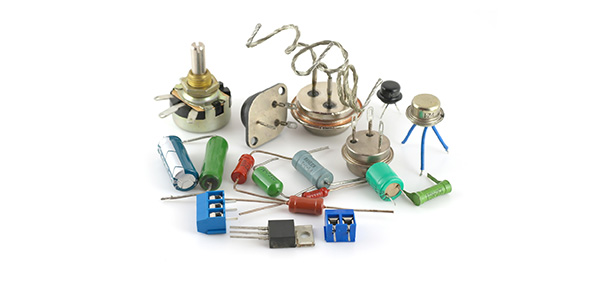# Modified Electricity Test

20 QuestionsSettings• 1.
Circuit with more than one path
• A.

Parallel

• B.

Series

• 2.
A material that current does not easily flow through
• A.

Conductor

• B.

Insulator

• 3.
Rapid movement of excess electrons from one place to another
• A.

Static electricity

• B.

Electric discharge

• 4.
Closed path through which a current can flow
• A.

Circuit

• B.

Voltage

• 5.
Buildup of electrical charges in one place.
• A.

Current electricity

• B.

Static electricity

• 6.
• A.

Static charge

• B.

Current

• 7.
Measure of how difficult it is for electrons to flow
• A.

Series

• B.

Resistance

• 8.
A material that current flows through easily
• A.

Conductor

• B.

Insulator

• 9.
A circuit with more than one path along which current can flow
• A.

Series

• B.

Parallel

• 10.
Place a checkmark beside all the following that are good conductors.  Assume water has minerals in it.
• A.

Dry skin

• B.

Water

• C.

Rubber

• D.

Iron

• E.

Copper

• F.

Styrofoam

• 11.
Place a checkmark beside all of the insulators.  Assume water has minerals in it.
• A.

Iron

• B.

Rubber

• C.

Dry skin

• D.

Copper

• E.

Styrofoam

• F.

Water

• 12.
A toaster is plugged into a 110-volt outlet.  What is the resistance of the toaster if the current in the toaster is 10 A?  Write your answer to the nearest 10th.  _____Ohms
• 13.
A hair dryer uses 1,000 W  of power when it is plugged into a 110-volt outlet.  What is the current in the hair dryer?  Write your answer to the nearest 10th.  ______A
• 14.
A lightbulb with a resistance of 10 ohms is connected to a battery.  If the current in the lightbulb is 0.10 A, what is the voltage of the battery?  Write your answer to the nearestr 10th.  ____ V
• 15.
Which of these things does a circuit need to work properly?
• A.

Fan

• B.

A power supply

• 16.
If a circuit is not complete
• A.

It will not work

• B.

It might work

• 17.
What do cells (batteries) do in a circuit
• A.

Nothing

• B.

Provide electricity

• 18.
Will the following series circuit work?
• A.

Yes, it is a complete circuit.

• B.

No, it is not a complete circuit.

• 19.
Will the following LED light light up?
• A.

Yes, it is a complete circuit

• B.

No, the switches are open

• 20.
Why do circuits need to be complete?
• A.

So that the electricity can travel all the way around

• B.

So the wires are all the same size